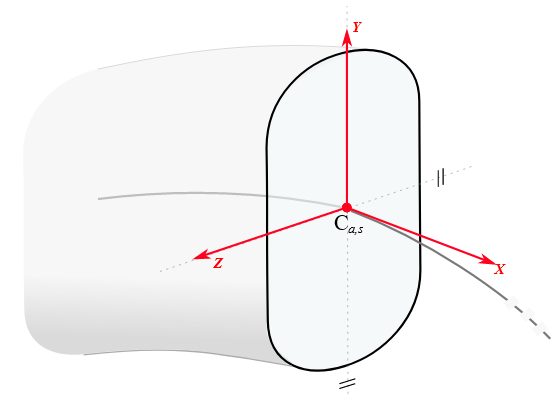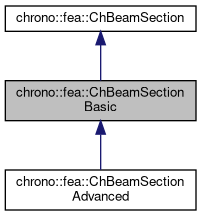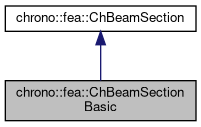chrono::fea::ChBeamSectionBasic Class Reference

## Description

Basic geometry for a beam section in 3D, along with basic material properties (zz and yy moments of inertia, area, Young modulus, etc.) This material can be shared between multiple beams.#include <ChBeamSection.h>

Inheritance diagram for chrono::fea::ChBeamSectionBasic:[legend]
Collaboration diagram for chrono::fea::ChBeamSectionBasic:[legend]

## Public Member Functions

void SetArea (const double ma)
Set the cross sectional area A of the beam (m^2)

double GetArea () const

void SetIyy (double ma)
Set the Iyy moment of inertia of the beam (for flexion about y axis) Note: some textbook calls this Iyy as Iz.

double GetIyy () const

void SetIzz (double ma)
Set the Izz moment of inertia of the beam (for flexion about z axis) Note: some textbook calls this Izz as Iy.

double GetIzz () const

void SetJ (double ma)
Set the J torsion constant of the beam (for torsion about x axis)

double GetJ () const

void SetKsy (double ma)
Set the Timoshenko shear coefficient Ks for y shear, usually about 0.8, (for elements that use this, ex. More...

double GetKsy () const

void SetKsz (double ma)
Set the Timoshenko shear coefficient Ks for z shear, usually about 0.8, (for elements that use this, ex. More...

double GetKsz () const

void SetAsRectangularSection (double width_y, double width_z)
Shortcut: set Area, Ixx, Iyy, Ksy, Ksz and J torsion constant at once, given the y and z widths of the beam assumed with rectangular shape.

void SetAsCircularSection (double diameter)
Shortcut: set Area, Ixx, Iyy, Ksy, Ksz and J torsion constant at once, given the diameter of the beam assumed with circular shape.

void SetDensity (double md)
Set the density of the beam (kg/m^3)

double GetDensity () const

void SetYoungModulus (double mE)
Set E, the Young elastic modulus (N/m^2)

double GetYoungModulus () const

void SetGshearModulus (double mG)
Set G, the shear modulus.

double GetGshearModulus () const

void SetGwithPoissonRatio (double mpoisson)
Set G, the shear modulus, given current E and the specified Poisson ratio.

void SetBeamRaleyghDamping (double mr)
Set the Rayleigh damping ratio r (as in: R = r * K ), to do: also mass-proportional term.

double GetBeamRaleyghDamping ()Public Member Functions inherited from chrono::fea::ChBeamSection
void SetDrawShape (std::shared_ptr< ChBeamSectionShape > mshape)
Set the graphical representation for this section. More...

std::shared_ptr< ChBeamSectionShapeGetDrawShape () const
Get the drawing shape of this section (i.e.a 2D profile used for drawing 3D tesselation and visualization) By default a thin square section, use SetDrawShape() to change it.

void SetDrawThickness (double thickness_y, double thickness_z)
Shortcut: adds a ChBeamSectionShapeRectangular for visualization as a centered rectangular beam, and sets its width/height. More...

Shortcut: adds a ChBeamSectionShapeCircular for visualization as a centered circular beam, and sets its radius. More...

void SetCircular (bool ic)
OBSOLETE only for backward compability More...

double Area

double Iyy

double Izz

double J

double G

double E

double density

double rdamping

double Ks_y

double Ks_z

## ◆ SetKsy()

 void chrono::fea::ChBeamSectionBasic::SetKsy ( double ma )
inline

Set the Timoshenko shear coefficient Ks for y shear, usually about 0.8, (for elements that use this, ex.

the Timoshenko beams, or Reddy's beams)

## ◆ SetKsz()

 void chrono::fea::ChBeamSectionBasic::SetKsz ( double ma )
inline

Set the Timoshenko shear coefficient Ks for z shear, usually about 0.8, (for elements that use this, ex.

the Timoshenko beams, or Reddy's beams)

The documentation for this class was generated from the following file:
• /builds/uwsbel/chrono/src/chrono/fea/ChBeamSection.h